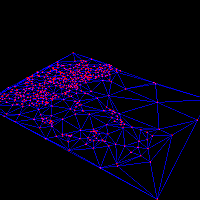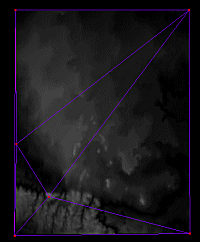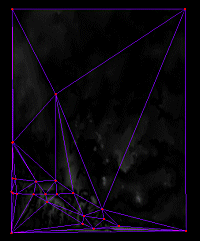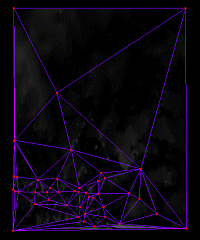Iterative TIN Introduction In a previous project on Delaunay Triangulation and Simplification, it was discovered that having control of the simplification process is extremely important for producing high quality efficient triangulations of terrain. The previous project's simplification scheme provided control for how much local error was admitted into the terrain, but no global error control and no control of the final polygon count. The iterative TIN algorithm, iTIN for short, was identified as the algorithm to correct these short comings, and this project is the result. The iTIN algorithm, also known as the "greedy-insertion" algorithm (see Garland & Heckbert), is a different approach to generating a triangulation than the previous projects. The previous projects used : An algorithm to sweep through the terrain's data samples, determining which ones to include based upon differences between that data sample and the most recently samples near it. No feedback from the resulting triangulation was used. A progressive meshing algorithm - which is the exact opposite of the Iterative TIN algorithm in that it decimates a large mesh by removing the edges that have the least impact on the mesh. The Iterative TIN algorithm is based on the concept that the triangulation can be iteratively generated, checking the triangulation between each vertex to determine where it should triangulate next. First the basic iTIN algorithm will be described as I have implemented it, then a few depth sections will be included to expand upon the triangulation, error metric, and error grid concepts. iTIN Algorithm The algorithm is extremely simple. Start with a basic triangulation, find the point, from the original terrain data, that when represented by that basic triangulation contains the most error, and add that point to the triangulation. (This eliminates the error at that point, but changes the potential error in the neighborhood around it.) Continue this process of finding the point with the maximum error and adding it to the triangulation until you have met a threshold for error or the number of polygons that you desire. Below is a bit of pseudo code for the algorithm:50 polygons250 polygons750 polygons150 polygons450 polygons1050 polygons ```Load the DEM file. Build a base triangulation using the four corners. Mark the entire error grid as dirty. ``` The above images are TINs generated by the algorithm for a portion of the Denver DEM. Notice how the algorithm is focusing triangulation on the mountainous region, leaving the areas with less error at lower triangulation levels. Click on the images for larger versions. ```while threshold condition is met (polys < threshold or error > threshold) Compute the error grid for the dirty region Find the point with the largest error. Insert the point in the triangulation. Compute the bounds of the triangles effected by the insertion. Mark the bounds as dirty in the error grid. ``` Obviously this is a simplification of the algorithm but this is pretty much it. Each of the operations, triangulation, building the error grid, and determining the error are independent of each other and various implementations can be used for them. The following sections describe the implementations that I have used for them. Triangulation To produce the TIN, there must be a triangulation algorithm. This triangulation algorithm does not need to know anything about what it is being used for but it does need to behave in a manner amenable to the the iTIN algorithm. The iTIN requires the ability to insert a point and then access the triangulation for intersections, then do the process again. For this project, the triangulation engine started with the Delaunay triangulation algorithm developed for theDelaunay Triangulation and Simplification project. Unfortunately, the insertion nature of the iTIN algorithm doesn't work well for that implementation. The previous implementation was designed for scenarios where all of the vertices of the triangulation were available at the start, and one pass was performed to produce the final triangulation. As described above, the iTIN algorithm needs to iteratively feed vertices to the triangulation, checking the intermediate triangulations as it proceeds to generate error metric values. Therefore, the Delaunay implementation had to be refactored for the new usage. This mostly effected what types of optimizations could be used in the algorithm and how some of the base triangulation work was performed. Error Metric The error metric used is a Local Error metric, which is just the distance between the triangulated terrain and the original DEM data. It allows us to concentrate the triangulation on areas of the terrain where the triangulated terrain would misrepresent the original terrain by the largest factor. To illustrate this, a DEM of Denver was selected. The DEM contains a mountainous region and then relatively placid areas. The algorithm was expected to concentrate the triangulation on the mountainous region. The above images illustrate that the algorithm did indeed do just this. It is hard to see in the images but the left most region of the DEM is mountainous and therefore requires more triangles to accurately represent the original terrain. The Local Error metric could be slightly improved upon by changing it to a more global error metric. The local metric eliminates the maxmimum error at the one data sample, but can potentially introduce quite a bit more error into a good triangulation by effecting neighboring points which may not have much error. A more global error metric would determine which region has the most error, then pick from the points within that region. This would reduce the significance of individual values which contain large amounts of error, which reside in a region which otherwise has little error. The nice part of the iTIN algorithm is that any error metric can be used, as it is an isolated component in the implementation. The triangulation is performed in UTM coordinates, and all error metrics are computed in the original height values. By doing the error calculations in UTM space, the error is accurate for the final triangulation as it is output in UTM coordinates. This allows specifying error thresholds in real world units. Error Grid The iTIN algorithm must store the error metric values for all of the sample points. It needs to access these with each iteration, such that it can compute the point with the largest error. In the implementation, a grid of error values that matches the DEM sample grid spacing is utilized. This error grid is updated by sampling the DEM grid and the triangulation for each point. It stores the difference between the two. The grid can locate the point with the maximum error, returning that point for the iTIN algorithm. The error grid maintains a dirty region which requires updating due to the previous insertion in the triangulation. It updates this dirty region before returning the next point with maximum error. Feature Insertion The iTIN algorithm works extremely well for scenarios where specific feature data points must be in the triangulation but control is desired on the size of the triangulation. In this case, the feature data points can be added to the triangulation before the algorithm begins. After this point, the algorithm and threshold metrics all work as usual. This guarantees the feature is included in the a well formed triangulation, without having to insert and cut triangles post-facto. Optimization The iTIN algorithm in its most naive implementation requires some optimization. The core of the algorithm doesn't directly require optimization, but the computation of the grid of error metric values does. Over 90% of the algorithm's time is spent in updating the error grid. And most of this time includes intersecting the current triangulation for the given bounding region. For this project, an optimization for the triangulation intersection was written and is discussed below. Triangulation Intersection Optimization As the number of triangles in the triangulation increases, we only want to test those triangles for intersection which could be in the vicinity of the intersection point. Therefore it was decided to include a spatial optimization to provide efficient access to the triangles in a given area. In this implementation, the triangulation engine divides the triangulation space into a grid of NxM cells. It maintains a list of all the triangles whose circumcircle intersects the cell. As the triangulation is updated, the lists in the cells are updated. When an intersection is requested, the algorithm finds the cell that contains the intersection to test and uses that list of triangles for the interseciton test. Though there is a bit of book keeping overhead, this optimization provides a good improvement in performance. The improvement on a 1200x1200 DEM, generating 500 polygons and using a 16x16 cell grid, is about 5x over a naive implementation. The reason for including triangles into cells based upon their circumcircle is so that the triangulation algorithm can be optimized by this same technique. It uses the circumcircles, and therefore it needs a list of all the triangles whose circumcircle could enclose a point. (At this time, the implementation does not use this optimization because the triangulation is already extremely fast.) This is clearly not the only way to improve this algorithm. A BREP (Boundary Representation) structure could probably serve the same purpose, but the triangulation engine does not currently use one. It also may be a bit more difficult with a BREP to determine which triangles have circumcircles which affect a given point, without having to test a larger number of triangles. Though, I am sure given a bit of thought an elegant solution could be done for this scenario also. One optimization is to eliminate doing triangle intersections completely, and move all of the error metric processing down onto the GPU. This is discussed in the next section. GPU Based Error Metric Computation To compute our error metric we need to update a large grid of values each iteration. A GPU is well suited for this type of work. The fact that we are also using triangles to compute our error metric makes it a perfect match. The following images show the triangulation process with the error metric superimposed. The displayed error metric is actually the metric for the previous iteration, so it can be shown that the new vertices are being placed at areas with large error (bright spots.) Here is an overview of how the GPU based error metric computation works. First, the original DEM is rendered into a pbuffer. The depth components are saved into a texture. Next, the current triangulation is rendered into the same pbuffer, and the depth components are saved into a different texture. Finally, both textures are rendered into the pbuffer, with a fragment program subtracting their components and performing an absolute value on them before writing out the value as the color information. The pbuffer is read back into system memory and used as the error grid. The process is rather simple but you need to be careful about some of the artifacts that may occur due to the differences in the rasterization of one large triangle and many small ones. Mostly edge artifacts which would erroneously encourage the subdivision of edge areas on the triangulation that truly do not need it. The images to the right show then triangulation as it progresses in the early stages. They show how the algorithm is inserting vertices where the largest error can be seen in the superimposed error metric.To further understand the images, the DEM Depth Map is included to the right. Notice in the DEM depth map that there is a ridge along the lower left corner and that the iTIN algorithm concentrates the triangulation in this region to minimize the error. Also notice that there are a few peaks out towards the right and that the iTIN quickly adds these peaks to the triangulation and then reduces the subsequent error that occurs when a peak is added to a triangulation.DEM Depth Map. Conclusions The iTIN algorithm is a nice simple algorithm which can lead to high quality terrains for given polygon counts. The ability to use different metrics for insertion, to stop triangulation based upon polygon count, and to control the actual error in the terrain are great assets to the algorithm.

glenn@raudins.com